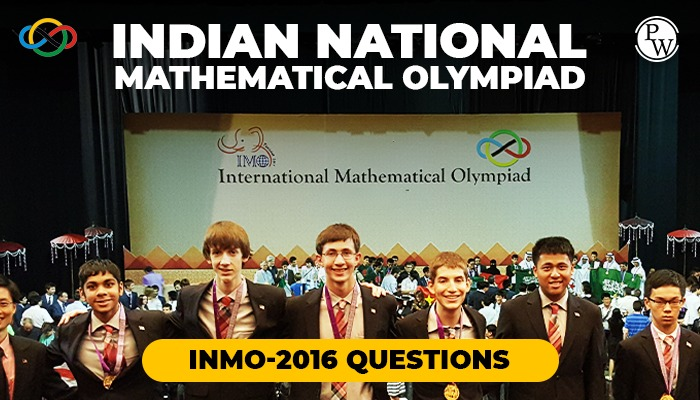# Indian National Mathematical Olympiad INMO-2016 Questions

The Mathematical Olympiad syllabus (regional, national and international) is pre-degree college mathematics. The Chapter covered is the arithmetic of integers, geometry, quadratic equations and expressions, trigonometry, co-ordinate geometry, precise mathematical system, permissions and combinations, factorization of polynomial, inequality, elementary combinatorics, probability theory and number theory, finite series, and complex numbers and elementary graph theory.The syllabus does not include both calculus and statistics. The main areas of concern are algebra, combinatorics, geometry, and number theory. The syllabus is sometimes spread across Class XI to Class XII levels, but the problems under each chapter involve a high level of difficulty and complexity. The difficulty level rises from RMO to INMO to IMO.

Download Indian National Mathematical Olympiad (INMO) 2016 Question Papers from Physics Wallah with step by step solution for all questions . practice from Physics Wallah maths study material read Class 10 Maths Notes.

### Find Below INMO previous year papers-2016 Question Papers